Next: An appraisal and some Up: The Binary Independence Model Previous: Probability estimates in practice   Contents   Index

## Probabilistic approaches to relevance feedback

We can use (pseudo-)relevance feedback, perhaps in an iterative process of estimation, to get a more accurate estimate of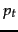. The probabilistic approach to relevance feedback works as follows:

1. Guess initial estimates ofand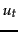. This can be done using the probability estimates of the previous section. For instance, we can assume thatis constant over all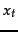in the query, in particular, perhaps taking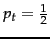.
2. Use the current estimates ofandto determine a best guess at the set of relevant documents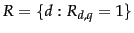. Use this model to retrieve a set of candidate relevant documents, which we present to the user.

3. We interact with the user to refine the model of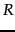. We do this by learning from the user relevance judgments for some subset of documents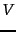. Based on relevance judgments,is partitioned into two subsets: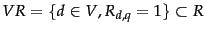and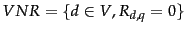, which is disjoint from.

4. We reestimateandon the basis of known relevant and nonrelevant documents. If the sets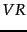and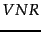are large enough, we may be able to estimate these quantities directly from these documents as maximum likelihood estimates: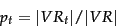(77)

(where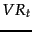is the set of documents incontaining). In practice, we usually need to smooth these estimates. We can do this by adding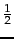to both the count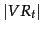and to the number of relevant documents not containing the term, giving: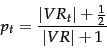(78)

However, the set of documents judged by the user () is usually very small, and so the resulting statistical estimate is quite unreliable (noisy), even if the estimate is smoothed. So it is often better to combine the new information with the original guess in a process of Bayesian updating . In this case we have: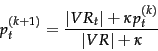(79)

Here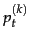is the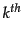estimate forin an iterative updating process and is used as a Bayesian prior in the next iteration with a weighting of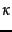. Relating this equation back to Equation 59 requires a bit more probability theory than we have presented here (we need to use a beta distribution prior, conjugate to the Bernoulli random variable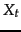). But the form of the resulting equation is quite straightforward: rather than uniformly distributing pseudocounts, we now distribute a total ofpseudocounts according to the previous estimate, which acts as the prior distribution. In the absence of other evidence (and assuming that the user is perhaps indicating roughly 5 relevant or nonrelevant documents) then a value of around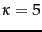is perhaps appropriate. That is, the prior is strongly weighted so that the estimate does not change too much from the evidence provided by a very small number of documents.

5. Repeat the above process from step 2, generating a succession of approximations toand hence, until the user is satisfied.

It is also straightforward to derive a pseudo-relevance feedback version of this algorithm, where we simply pretend that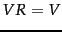. More briefly:

1. Assume initial estimates forandas above.
2. Determine a guess for the size of the relevant document set. If unsure, a conservative (too small) guess is likely to be best. This motivates use of a fixed size setof highest ranked documents.

3. Improve our guesses forand. We choose from the methods of and 79 for re-estimating, except now based on the setinstead of. If we let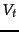be the subset of documents incontainingand use addsmoothing , we get: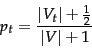(80)

and if we assume that documents that are not retrieved are nonrelevant then we can update ourestimates as: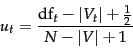(81)

4. Go to step 2 until the ranking of the returned results converges.

Once we have a real estimate forthen the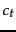weights used in the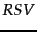value look almost like a tf-idf value. For instance, using Equation 73, Equation 76, and Equation 80, we have: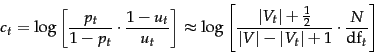(82)

But things aren't quite the same: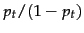measures the (estimated) proportion of relevant documents that the term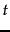occurs in, not term frequency. Moreover, if we apply log identities: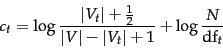(83)

we see that we are now adding the two log scaled components rather than multiplying them.

Exercises.

• Work through the derivation of Equation 74 from and 3()I .

• What are the differences between standard vector space tf-idf weighting and the BIM probabilistic retrieval model (in the case where no document relevance information is available)?

• Letbe a random variable indicating whether the termappears in a document. Suppose we have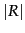relevant documents in the document collection and that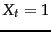in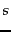of the documents. Take the observed data to be just these observations offor each document in. Show that the MLE for the parameter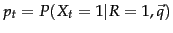, that is, the value forwhich maximizes the probability of the observed data, is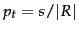.

• Describe the differences between vector space relevance feedback and probabilistic relevance feedback.Next: An appraisal and some Up: The Binary Independence Model Previous: Probability estimates in practice   Contents   Index